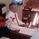# Indicator: Volatility Quality Index [VQI]

Dilihat 10126 kali
Volatility Quality Index (VQI), by Thomas Stridsman, points out the difference between bad and good volatility in order to identify better trade opportunities in the market.

This plots 3 lines:
- Red line is the VQI (actually, sum of VQI).
- Green line is the 9-period SMA of sum_of_VQI.
- Orange line is the 200-period SMA of sum_of_VQI.

Stridsman suggested to buy when VQI has increased in the previous 10 bars (use the SMAs ) and sell when it has decreased in the previous 10 bars. IMO , use this with your other indicators as a confirmation signal.

To use this indicator in your charts, click on "Share" button (top right on the chart). Click on "Make it mine" button on the dialog that pops up. Now, you will have a copy of this chart with the indicator's source code in it. Click on "{}" to open the source code of VQI_LB and save it to your custom scripts section.
```//
// @author LazyBear
// @credits Thomas Stridsman - http://www.3pips.com/volatility-quality-index-vq/
// If you use this code in its original/modified form, do drop me a note.
//
study("Volatility Quality Index [LazyBear]", shorttitle="VQI_LB")
length_slow=input(9, title="Fast EMA Length")
length_fast=input(200, title="Slow EMA Length")
vqi_t=iff((tr != 0) and ((high - low) != 0) ,(((close-close)/tr)+((close-open)/(high-low)))*0.5,nz(vqi_t))
vqi = abs(vqi_t) * ((close - close + (close - open)) * 0.5)
vqi_sum=cum(vqi)
plot(vqi_sum, color=red, linewidth=2)
plot(sma(vqi_sum,length_slow), color=green, linewidth=2)
plot(sma(vqi_sum,length_fast),color=orange, linewidth=2)
```
List of my free indicators: http://bit.ly/1LQaPK8
List of my indicators at Appstore: http://blog.tradingview.com/?p=970

## KomenDid you ever experiment with a "volatility quality zero line" Is that indicator similar to this one; if so can it be converted? Thanks
BalasThanks! Works really well. Short Length trigger and long length trigger
BalasQuantitativeExhaustion
I have sent you a pm reg posting some real life usecases here. Please let me know what you think.
BalasLazyBear
Type : Indicator, Name : Volatility Quality Index
Variables:
VQI(0),
SumVQI(0);

If TrueRange <> 0 and (High - Low) <> 0 Then
VQI = ((Close - Close) / TrueRange + (Close - Open) / (High - Low)) * 0.5
Else
VQI=VQI;
VQI = AbsValue(VQI) * ((Close - Close + (Close - Open)) * 0.5);
SumVQI = SumVQI + VQI;
Plot1(SumVQI,"");
Plot2(Average(SumVQI,9),"");
Plot3(Average(SumVQI,200),"");

try plugging in this ... I get a much different look
BalasQuantitativeExhaustion
This is the same. Plz check ur pm.
Balas